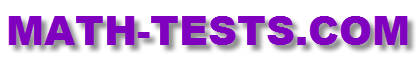#This 3rd Grade Multiplication Test is a great online activity for elementary students to check their understanding of basic multiplication facts.

The quiz has ten problems: seven multiple choice, and three drag and drop/matching problems.
Third grade students will use models and arrays to multiply numbers. Students will also solve multiplication problems by viewing them as repeated addition.

This test is based on the following Common Core Standards:

CCSS.Math.Content.3.OA.A.1
Interpret products of whole numbers, e.g., interpret 5 x 7 as the total number of objects in 5 groups of 7 objects each. For example, describe a context in which a total number of objects can be expressed as 5 x 7

CCSS.Math.Content.3.OA.A.4
Determine the unknown whole number in a multiplication or division equation relating three whole numbers. For example, determine the unknown number that makes the equation true in each of the equations 8 x ? = 48,

CCSS.Math.Content.3.OA.C.7
Fluently multiply and divide within 100, using strategies such as the relationship between multiplication and division (e.g., knowing that 8 x 5 = 40, one knows 40 ÷ 5 = 8) or properties of operations. By the end of Grade 3, know from memory all products of two one-digit numbers.